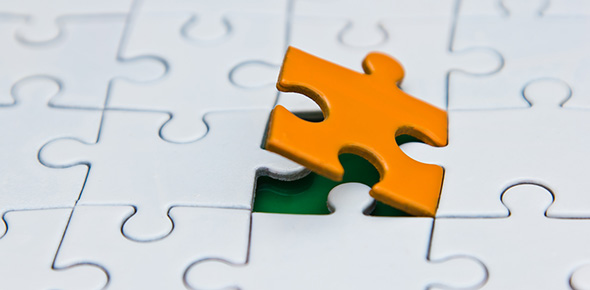# Primary Mathematics Level 6 (P6) Placement Assessment

14 Questions | Total Attempts: 1004Settings• 1.
Find the value of the expression when n = 8
• 2.
Amal is x years old. Bela is 4 times as old as Amal. Camila is 3 years older than Bela. (a) Express Camila's age in years in terms of x (b) If Amal is 2 years old, how old is Camila?
• A.

(a) 4x (b) 8 years

• B.

(a) x + 3 (b) 5 years

• C.

(a) 4 + 3x (b) 10 years

• D.

(a) 4x + 3 (b) 11 years

• E.

(a) 4x + 3x (b) 14 years

• 3.
(a) Express Andy's money as a fraction of Bob's money (b) If Andy has \$12 more than Bob, how much money do they have altogether?
• A.

(a) 6/5 (b) \$142

• B.

(a) 5/6 (b) \$138

• C.

(a) 5/6 (b) \$148

• D.

(a) 6/5 (b) \$138

• E.

(a) 6/5 (b) \$148

• 4.
Mrs. Khan mixed meat with potatoes in the ratio of 5 : 3 to make 4 kg of meat loaf. How much meat did she use?
• A.

1 kg 500 g

• B.

2 kg 500 g

• C.

2 kg 800 g

• D.

3 kg

• E.

3 kg 200 g

• 5.
A fuel tank of a car is 80% full. After traveling some distance, only 30% of that fuel is left. The tank is then filled to its full capacity by putting in 19 liters. What is the full capacity of the tank?
• A.

22 liters

• B.

24 liters

• C.

25 liters

• D.

30 liters

• E.

32 liters

• 6.
A cyclist took 3 h to cycle from Town X to Town Y. His average speed was 12 km/h. If his average speed were increased by 3 km/h, how much time would he then take for the journey?
• A.

2 h

• B.

2 h 36 min

• C.

2 h 30 min

• D.

2 h 24 min

• E.

2 h 15 min

• 7.
The figure is made using three half circles. Find its area and perimeter.
• A.

Area = 1824 cm square, Perimeter = 240 cm

• B.

Area = 1848 cm square, Perimeter = 264 cm

• C.

Area = 1788 cm square, Perimeter = 256 cm

• D.

Area = 1818 cm square, Perimeter = 254 cm

• E.

Area = 1856 cm square, Perimeter = 246 cm

• 8.
Anya is riding a bicycle with tires that have a radius of 28 cm. If the tires have made 1,250 revolutions since she started, how far has she traveled?
• A.

2.2 km

• B.

2.15 km

• C.

2.1 km

• D.

2.05 km

• E.

2 km

• 9.
This pie chart represents the use of monthly income. (a) What percentage of the monthly income is saved? (b) If \$264 is saved, how much is the monthly income?
• A.

(a) 11% (b) \$2400

• B.

(a) 10% (b) \$2640

• C.

(a) 15% (b) \$1760

• D.

(a) 12% (b) \$2200

• E.

(a) 18% (b) \$1467

• 10.
The figure is not drawn to scale. ABCD is a parallelogram. BEF and CDF are straight lines. BC = CF.
• A.

32 degrees

• B.

31 degrees

• C.

30 degrees

• D.

28 degrees

• E.

29 degrees

• 11.
A car left Town A at 10:00 a.m. and travelled towards Town B at the average speed of 70 km/h. At the same time a truck left Town B and travelled towards Town A over the same road at an average speed of 50 km/h. If the distance between Town A and Town B is 420 km, at what time would the car and the truck pass each other?
• A.

12:30 pm

• B.

1:00 pm

• C.

1:30 pm

• D.

1:45 pm

• E.

2:00 pm

• 12.
Last month Dara and Maryam saved some money in a ratio of 3 : 5. This month they saved an additional \$154 together, and Dara now has three times as much money as he had last month, while Maryam has two times as much as she had last month. How much money did they save last month?
• A.

\$114

• B.

\$112

• C.

\$118

• D.

\$116

• E.

\$110

• 13.
The base of a rectangular tank measures 50 cm by 40 cm. It contains 60 litres of water when it is full. Find the height of the tank.
• A.

40 cm

• B.

35 cm

• C.

45 cm

• D.

50 cm